## Source Transformation Technique:

Sometimes in the complex network, both the energy sources may be present. But in loop analysis, all the energy sources should be preferably voltage sources and in node analysis, all the energy sources should be preferably current sources. The source transformation technique is useful in converting voltage to current source transformation and current to voltage source transformation.

The replacement of a particular energy source by an equivalent source is called Source Transformation. A non ideal voltage source and a non ideal current source are said to be equivalent to each other if at all the terminals, the sources show same volt-ampere characteristics. So while doing Source Transformation Technique, the following conditions must be satisfied,

1. The open circuit voltages at their terminals must be equal.
2. The short circuit current at their terminals must be equal.

Consider circuit shown in Fig. 1.25 (a).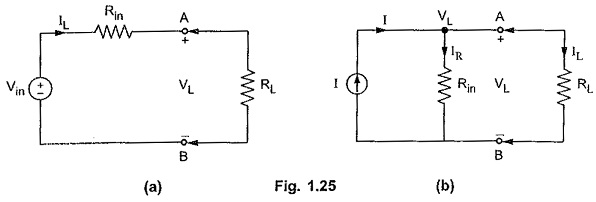Applying KVL in the Fig. 1.25 (b),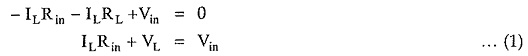Now applying KCL at node 1 in the Fig. 1.25 (b).

I = IR + IL

Now circuit shown in the Fig 1.25 (b) is equivalent circuit for the circuit shown in Fig. 1.25 (a)

where

• I = Vin/Rin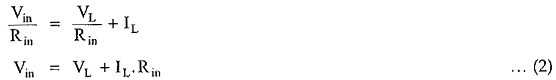So equations (1) and (2) are the same equations. Thus we can conclude that, a voltage source V in series with resistance R can be converted to a current source of value (V ∕ R) which is in parallel with resistor R.

Conversely, a current source I in parallel with resistance R can be converted to a voltage source of value (IR) which is in series with R.

Thus if in a given network there exists a voltage source along with an impedance Z in series with it, it can be converted to an equivalent current source as shown in the Fig. 1.25 (c).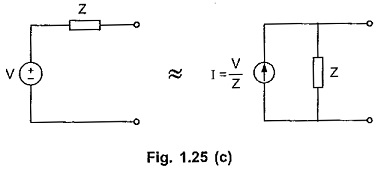The current source is given by, I = V ∕ Z

while the same impedance Z now exists in shunt with the current source.

Similarly if there exists a current source with an impedance Z in shunt with it, it can be replaced by an equivalent voltage source given by,

V = IZ

with same impedance Z in series with the voltage source, as shown in the Fig. 1.25 (d).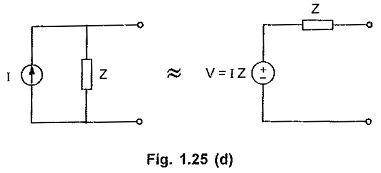The direction of current of equivalent current source is always from -ve to + ve, internal to the source. While converting current source to voltage source, polarities of voltage is always as +ve terminal at top of arrow and -ve terminal at bottom of arrow, as direction of current is from -ve to +ve, internal to the source. This ensures that current flows from positive to negative terminal in the external circuit.

Note the directions of Source Transformation Technique shown in the Fig. 1.26 (a), (b), (c) and (d).

Scroll to Top